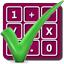#### Arithmetic of Computers

#### from Tenscope Limited

By using this site, you are accepting "session" cookies, as set out in the site's Privacy Policy
Cookies are also used to remember which page of the book you last viewed, so that when you revisit the site you automatically return to the last page you visited.

### Lesson 6

#### Page 191

Your answer :
No.
You are correct.
So long as we are agreed on the number for the base of our number system, we may use any number we please.
A number system based on powers of 8 finds considerable use around digital computers. This system is called the octal system (Latin octo = eight). To introduce the basic ideas in the octal system, let’s consider first the following question:
What decimal number is represented by the expression
1(82) + 3(81) + 2(80)?
Answer :
1(82) + 3(81) + 2(80) = 89.
1(82) + 3(81) + 2(80) = 90.
1(82) + 3(81) + 2(80) = 104.

Answer to Self-Test Question 1, Lesson 6 :
a. 262; b. 28; c. 346; d. 136.